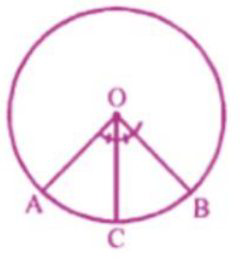# ML Aggarwal Solutions Class 9 Mathematics Solutions for Circle Exercise 15.2 in Chapter 15 - Circle

Question 2 Circle Exercise 15.2

A and B are points on a circle with center O. C is a point on the circle such that OC

bisects ∠AOB, prove that OC bisects the arc AB.

Given: in a given circle with center O, A

And B is Two points on the circle. C i

another point on the circle such that

∠AOC = ∠BOCTo prove : arc AC = arc BC

Proof: OC is the bisector of ∠AOB

Or ∠AOC = ∠BOC

But these are the angle subtended by the

arc AC and BC

arc AC = arc BC.

Video transcript
"hello and welcome my dear students i am rishabh quran the man student on lido and i'm here a new question it says a and b are points on a circle with center row c is a point on the circle such that oc bisects angle aob prove that oc bisects the arc a b so without wasting much time let's have a look at the solution we will first look at the given part so we have been given a circle let's center o in which a and b are two points on the circle also we have been given that c is another point such that angle aoc is equals to angle boc what we are required to prove here let our ac is equals to our pc let's look at the proof so we know that oc is the bisector of angle aob right so which means that angle aoc is equals to angle boc right but these are the angles obtained by the arc ac and bc so for subtended i am writing suv only which means that arc ac equals to r bc i hope my students got this question well do not forget to hit the subscribe button you can also share your doubts in the comment section see you next time bye"

Lido

Courses

Teachers

Book a Demo with us

Syllabus

Maths
CBSE
Maths
ICSE
Science
CBSE

Science
ICSE
English
CBSE
English
ICSE
Coding

Terms & Policies

Selina Question Bank

Maths
Physics
Biology

Allied Question Bank

Chemistry
Connect with us on social media!